Electric and Magnetic Fields Chapters 17 21 Electric

• Slides: 33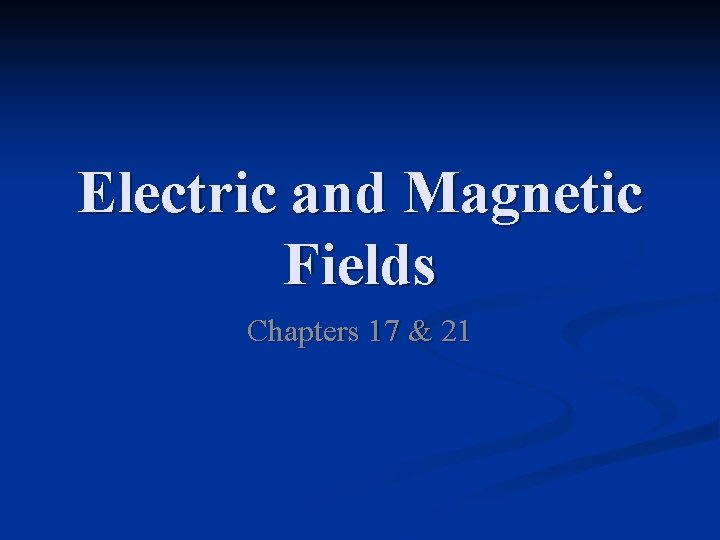Electric and Magnetic Fields Chapters 17 & 21Electric Field n Electric force, like gravitational force, is a field force n n Remember: Field forces can act through space even when there is no physical contact between the objects involved A charged object has an electric field in the space around it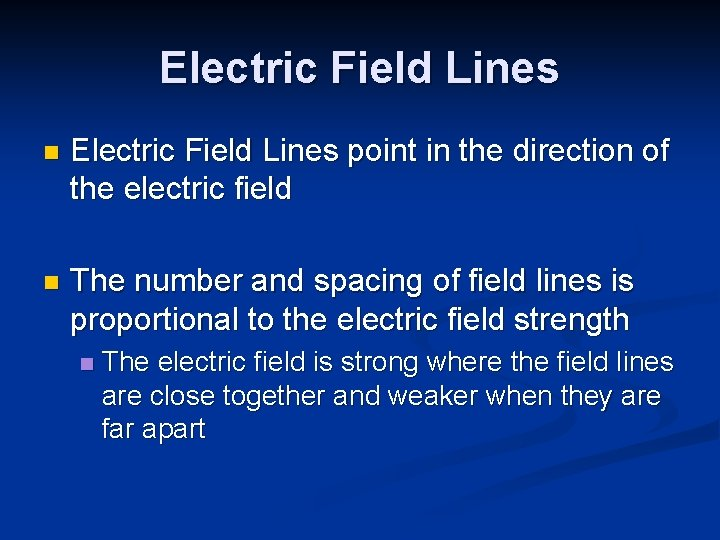Electric Field Lines n Electric Field Lines point in the direction of the electric field n The number and spacing of field lines is proportional to the electric field strength n The electric field is strong where the field lines are close together and weaker when they are far apart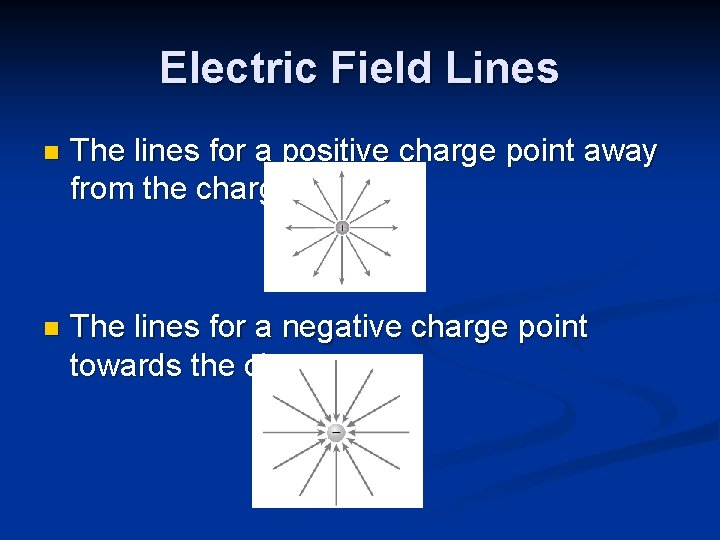Electric Field Lines n The lines for a positive charge point away from the charge n The lines for a negative charge point towards the charge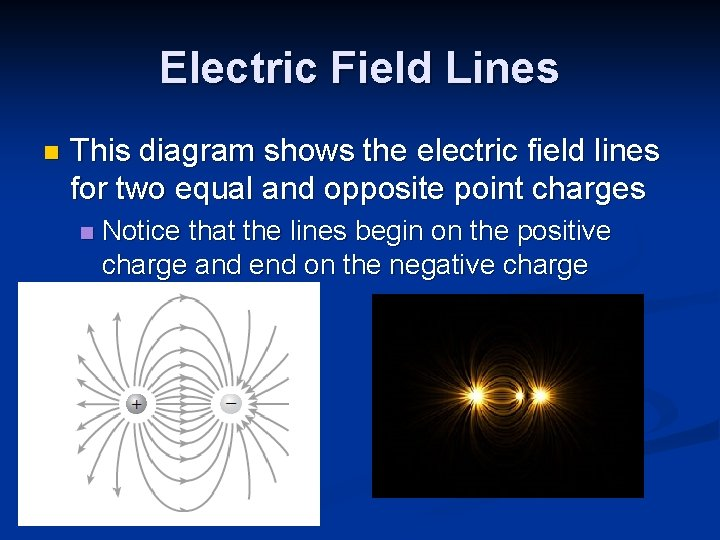Electric Field Lines n This diagram shows the electric field lines for two equal and opposite point charges n Notice that the lines begin on the positive charge and end on the negative charge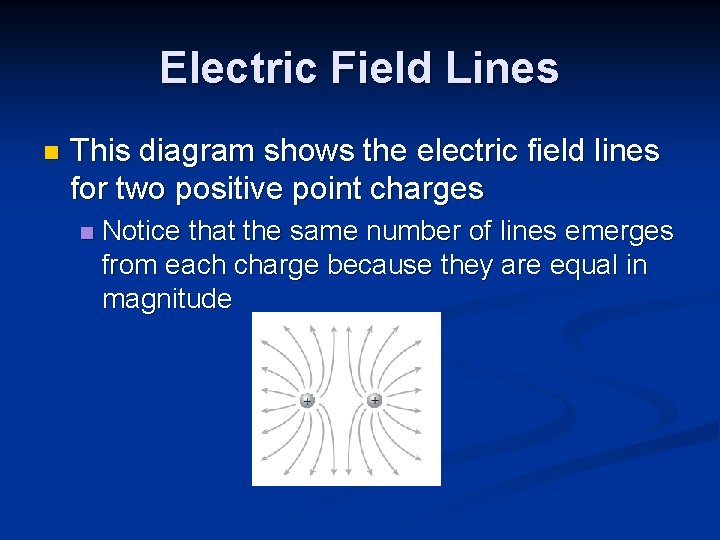Electric Field Lines n This diagram shows the electric field lines for two positive point charges n Notice that the same number of lines emerges from each charge because they are equal in magnitude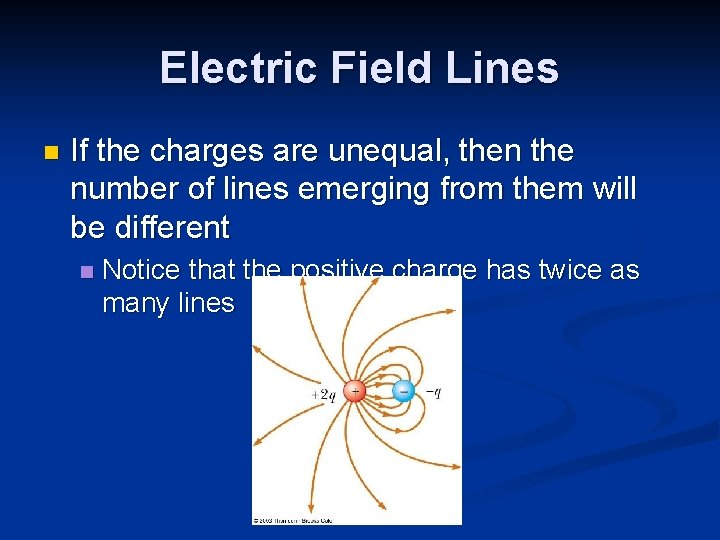Electric Field Lines n If the charges are unequal, then the number of lines emerging from them will be different n Notice that the positive charge has twice as many linesCalculating Electric Field Strength n The equation for the electric field produced by a point charge is: n n n Kc=9 x 109 Nm 2/C 2 , r is the distance from the charge and q is the charge producing the field The unit for E is N/C Electric field strength is a vector!! n n If q is positive, then E is directed away from q If q is negative, then E is directed toward q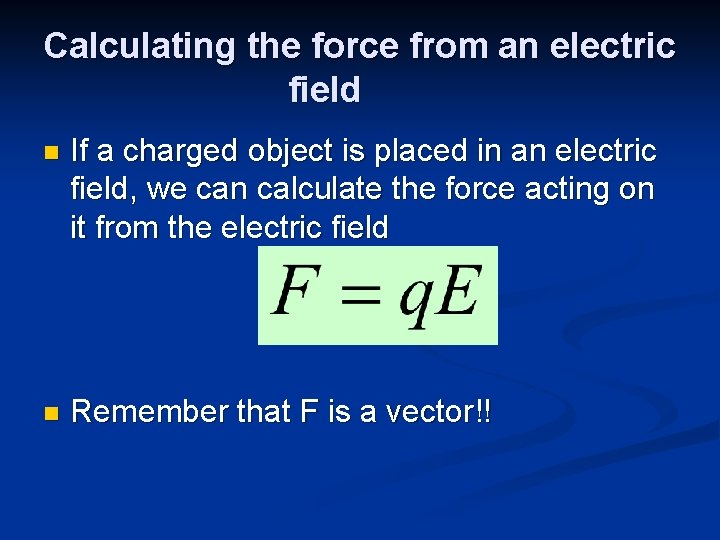Calculating the force from an electric field n If a charged object is placed in an electric field, we can calculate the force acting on it from the electric field n Remember that F is a vector!!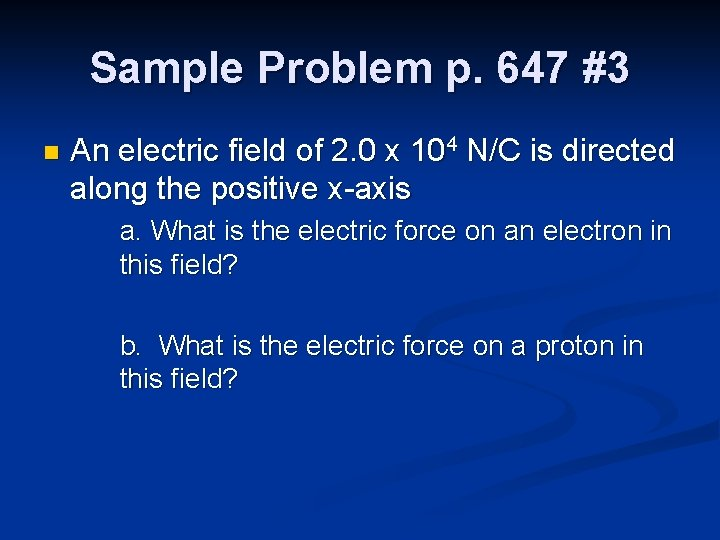Sample Problem p. 647 #3 n An electric field of 2. 0 x 104 N/C is directed along the positive x-axis a. What is the electric force on an electron in this field? b. What is the electric force on a proton in this field?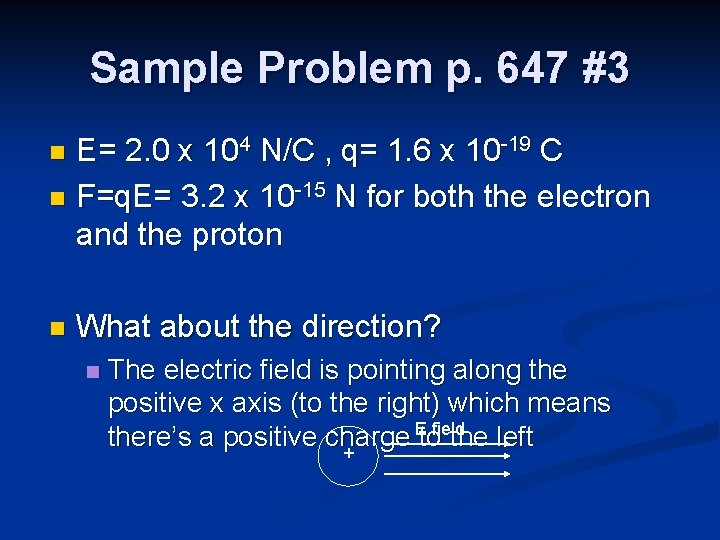Sample Problem p. 647 #3 E= 2. 0 x 104 N/C , q= 1. 6 x 10 -19 C n F=q. E= 3. 2 x 10 -15 N for both the electron and the proton n n What about the direction? n The electric field is pointing along the positive x axis (to the right) which means E field there’s a positive charge to the left +For the proton n Since the electric field is pointing to the right, if you put a proton in it, the proton will want to move away towards the right and the direction of the force on it will be to F the right + + n Answer: 3. 2 x 10 -15 N along the positive x axis (to the right)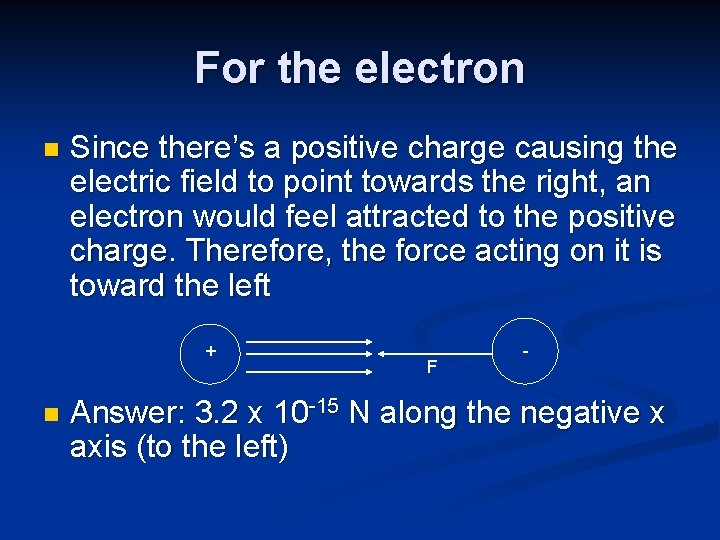For the electron n Since there’s a positive charge causing the electric field to point towards the right, an electron would feel attracted to the positive charge. Therefore, the force acting on it is toward the left + n F - Answer: 3. 2 x 10 -15 N along the negative x axis (to the left)Sample Problem p. 656 #38 n Find the electric field at a point midway between two charges of +30 n. C and 60 n. C separated by a distance of 30. 0 cm +30 n. C +60 n. C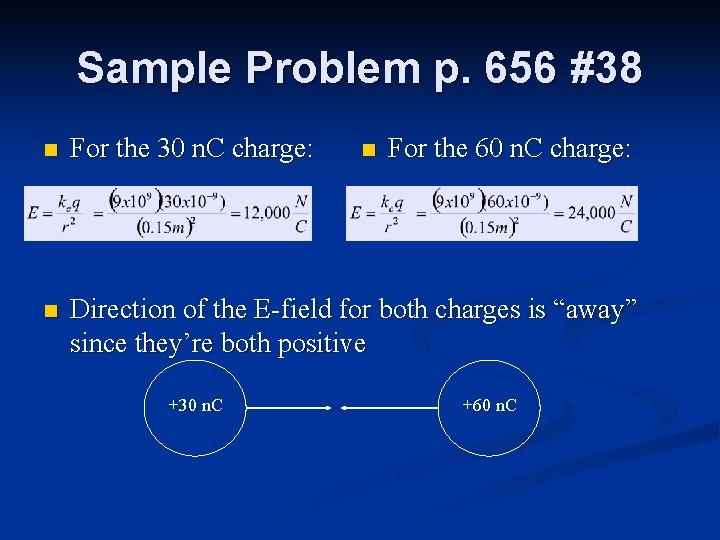Sample Problem p. 656 #38 For the 60 n. C charge: n For the 30 n. C charge: n Direction of the E-field for both charges is “away” since they’re both positive +30 n. C n +60 n. C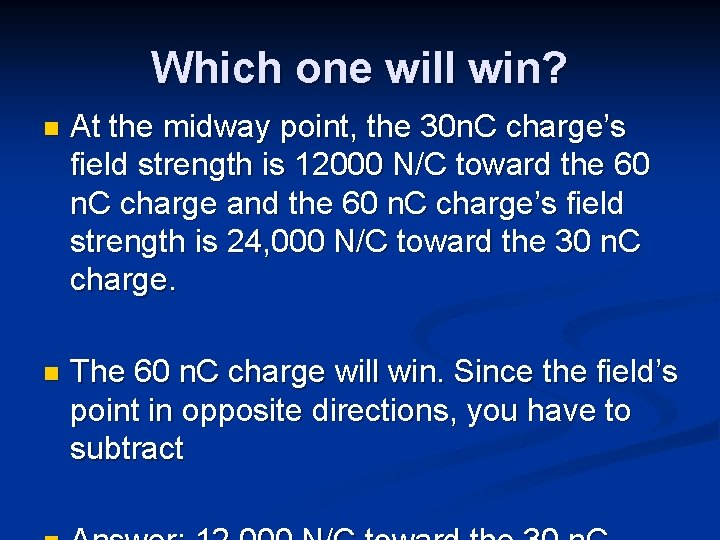Which one will win? n At the midway point, the 30 n. C charge’s field strength is 12000 N/C toward the 60 n. C charge and the 60 n. C charge’s field strength is 24, 000 N/C toward the 30 n. C charge. n The 60 n. C charge will win. Since the field’s point in opposite directions, you have to subtract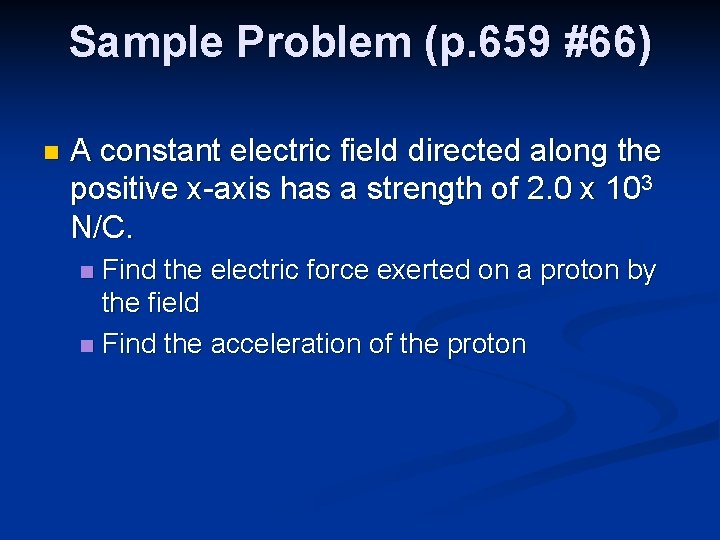Sample Problem (p. 659 #66) n A constant electric field directed along the positive x-axis has a strength of 2. 0 x 103 N/C. Find the electric force exerted on a proton by the field n Find the acceleration of the proton n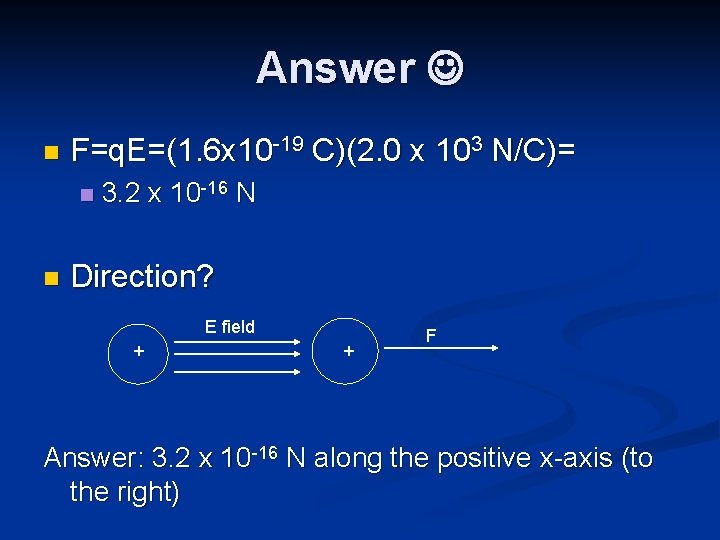Answer n F=q. E=(1. 6 x 10 -19 C)(2. 0 x 103 N/C)= n n 3. 2 x 10 -16 N Direction? E field + + F Answer: 3. 2 x 10 -16 N along the positive x-axis (to the right)Answer n B. What is the acceleration? n Ask Newton! F=ma n a = F/m= 3. 2 x 10 -16 N/1. 6 x 10 -27 kg n a= 2 x 1011 m/s 2 along the positive x axis n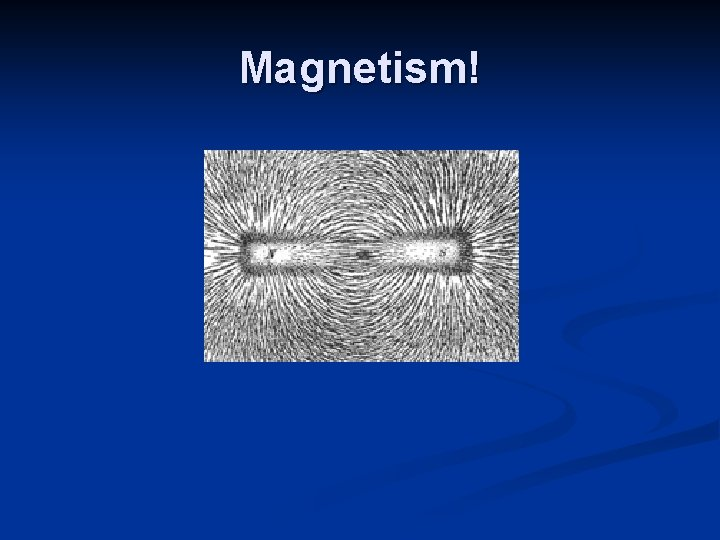Magnetism!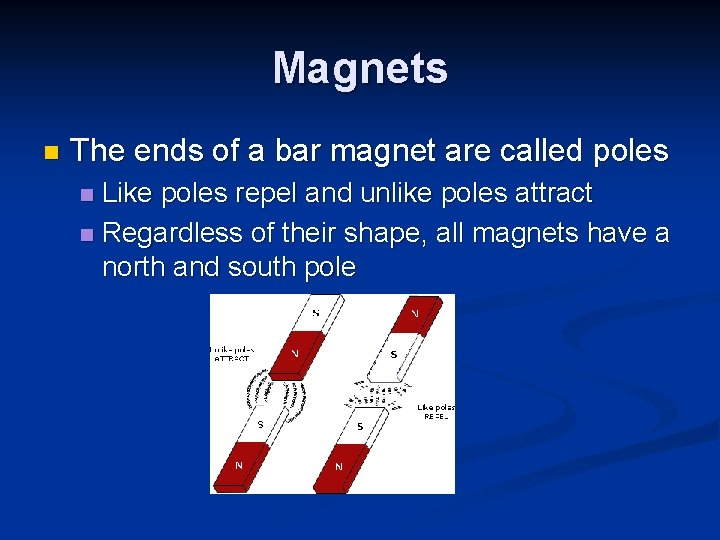Magnets n The ends of a bar magnet are called poles Like poles repel and unlike poles attract n Regardless of their shape, all magnets have a north and south pole n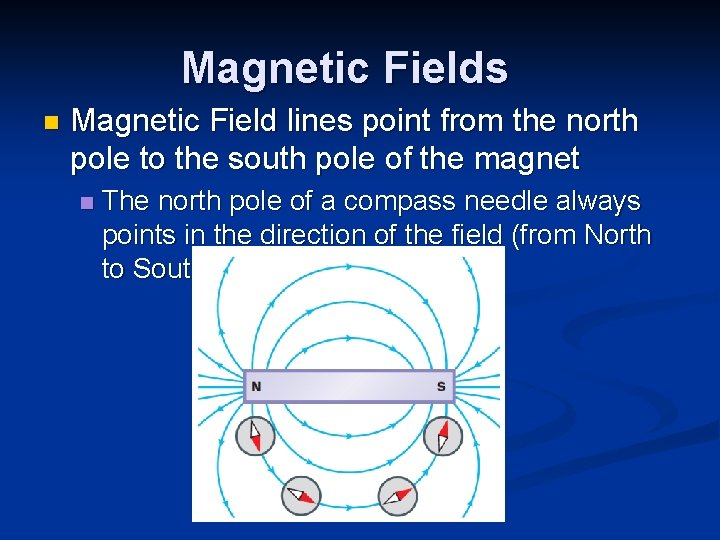Magnetic Fields n Magnetic Field lines point from the north pole to the south pole of the magnet n The north pole of a compass needle always points in the direction of the field (from North to South)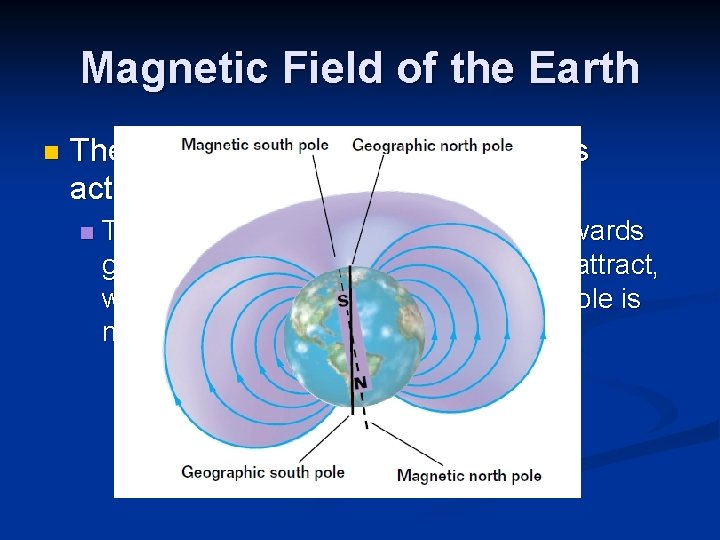Magnetic Field of the Earth n The Earth’s geographic North pole is actually the magnetic south pole n The north pole of a compass points towards geographic north and since opposites attract, we know that the Earth’s geographic pole is magnetic south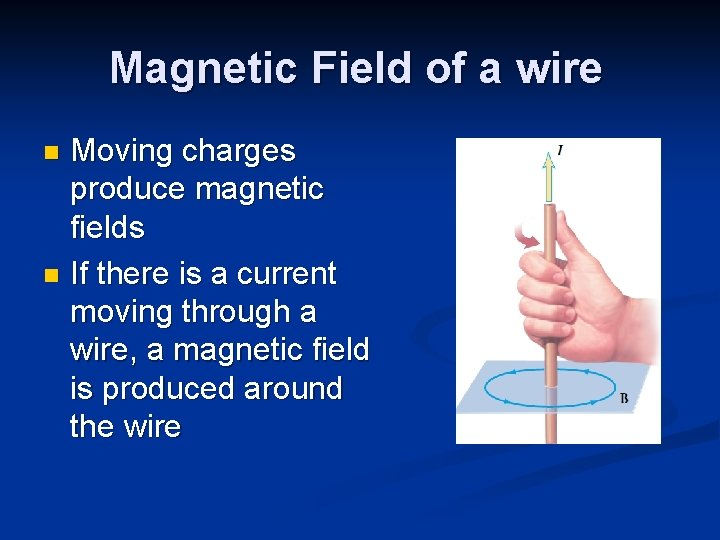Magnetic Field of a wire Moving charges produce magnetic fields n If there is a current moving through a wire, a magnetic field is produced around the wire n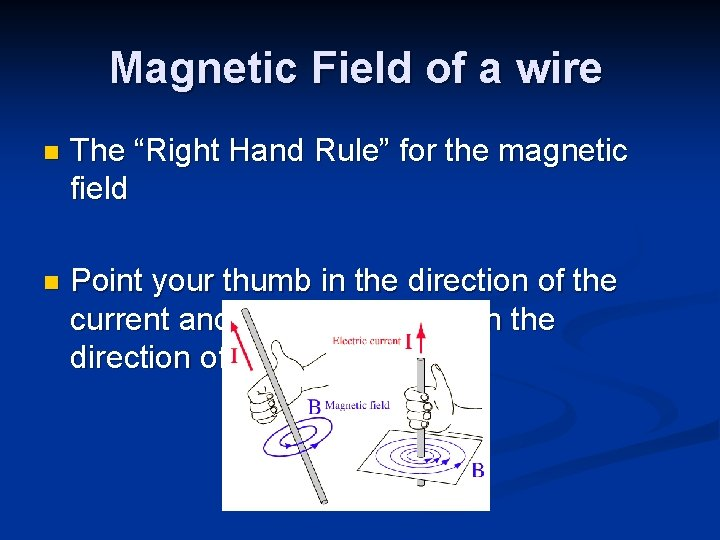Magnetic Field of a wire n The “Right Hand Rule” for the magnetic field n Point your thumb in the direction of the current and curl your fingers in the direction of the field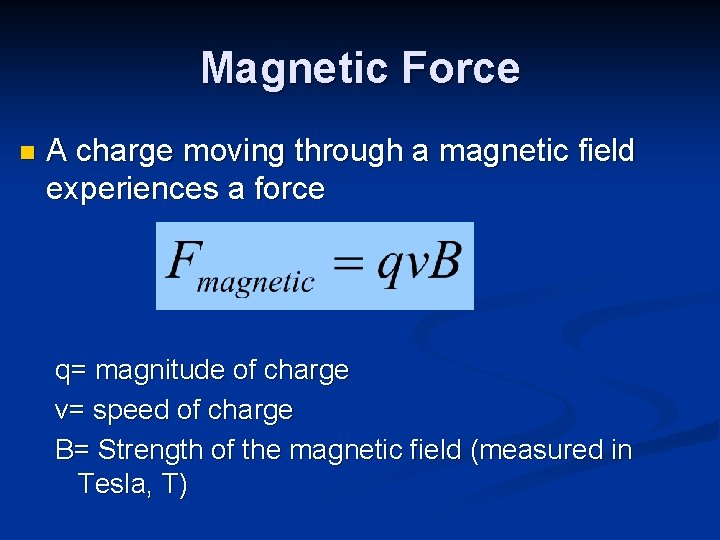Magnetic Force n A charge moving through a magnetic field experiences a force q= magnitude of charge v= speed of charge B= Strength of the magnetic field (measured in Tesla, T)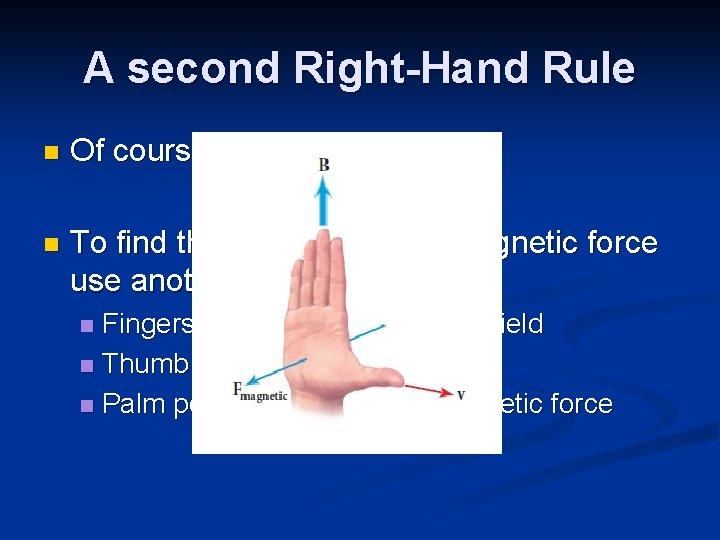A second Right-Hand Rule n Of course, force is a vector! n To find the direction of the magnetic force use another right hand rule Fingers point in direction of the field n Thumb points in direction of v n Palm points in direction of magnetic force nConventions for direction of field WARNING: The right Direction of Field Symbol Into the page X Out of the page hand rule is for the direction of the force acting on a POSITIVE CHARGE. To find the direction of the force acting on a negative charge, you’ll have to use the rule and change the sign!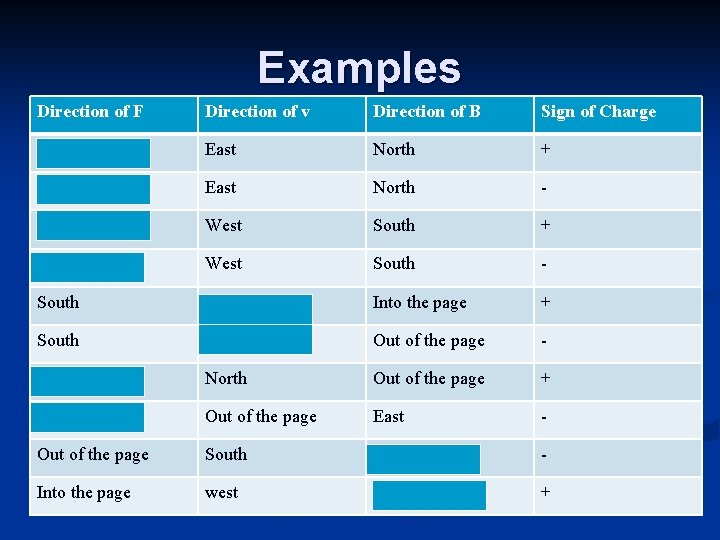Examples Direction of F Direction of v Direction of B Sign of Charge Out of the page East North + Into the page East North - Out of the page West South + Into the page West South - South West Into the page + South West Out of the page - East North Out of the page + South Out of the page East - Out of the page South West - Into the page west North +Sample Problem p. 775 #2 (edited) n A proton traveling to the right along the xaxis enters a region where there is a magnetic field of 2. 5 T directed north. If the proton experiences a force of 3. 2 x 10 -12 N, find the speed of the proton. What is the direction of the force exerted on the proton?The speed of the proton n What’s the direction of F? Use the RHR!! v is east, B is north…F is…. n Out of the page! n If it was an electron, the force would be into the page! n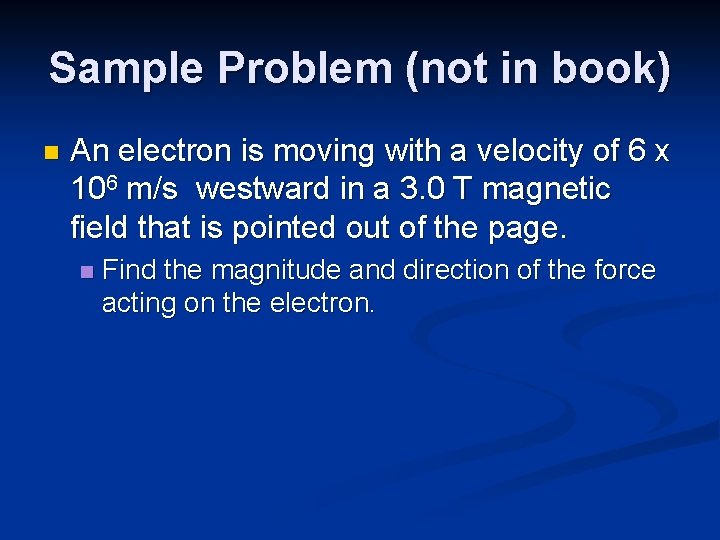Sample Problem (not in book) n An electron is moving with a velocity of 6 x 106 m/s westward in a 3. 0 T magnetic field that is pointed out of the page. n Find the magnitude and direction of the force acting on the electron.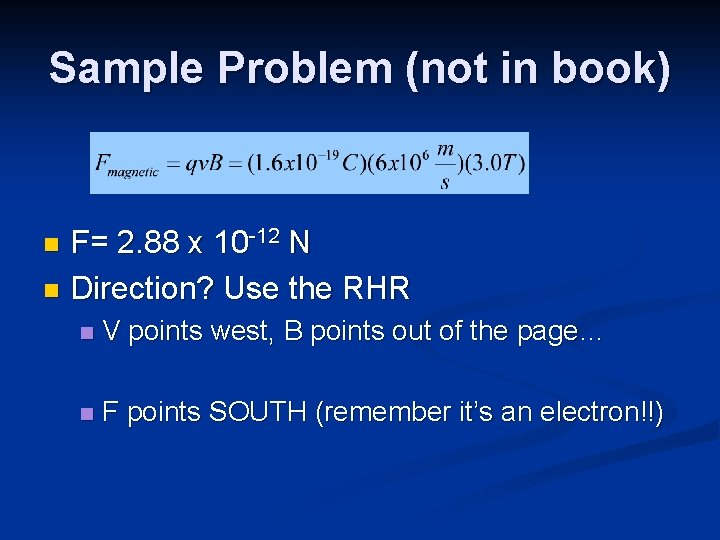Sample Problem (not in book) F= 2. 88 x 10 -12 N n Direction? Use the RHR n n V points west, B points out of the page… n F points SOUTH (remember it’s an electron!!)